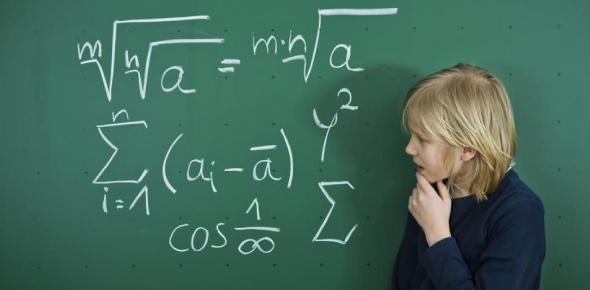# Could You Pass This Math Exam? MCQ Quiz

19 Questions | Attempts: 738Settings.

• 1.
Which of the following is an arithmetic sequence?
• A.

1, 2, 4, 7, 13, ...

• B.

1, 2, 3, 6, 9 ...

• C.

1, 2, 4, 6, 8 ...

• D.

1, 2, 4, 8, 16 ...

• 2.
A best-fit line drawn on a scatter plot _______________ .
• A.

Approximates the linear relationship of data points

• B.

Always has a positive slope

• C.

Must be drawn through the first and last data points

• D.

Is the line that goes through every data point

• 3.
The scatter plot below shows the women's winning marathon times and the high temperatures on the marathons days. Which predication equation best shows how marathon times and temperature are related?
• A.

Y = - (1/8)x + 140

• B.

Y = (1/8)x - 140

• C.

Y = (1/8)x + 140

• D.

Y = 140

• 4.
A best-fit line is drawn on a scatter plot showing the average daily temperature and the number of people at a public swimming pool. The slope of the line would probably be ________________.
• A.

Zero

• B.

Undefined

• C.

Negative

• D.

Positive

• 5.
The correlation seen in the graph below would best described as:
• A.

High positive correlation

• B.

Low positive correlation

• C.

High negative correlation

• D.

Low negative correlation

• 6.
For 10 days, Taylor kept a record of the number of hours he spent listening to music. The information is shown in the table below.  Day 1 2 3 4 5 6 7 8 9 10 Hours 9 3 2 6 8 6 10 4 5 2 Which scatter plot shows Taylor's data graphically?
• A.
• B.
• C.
• D.
• 7.
A scatterplot is _________________________.
• A.

A line graphed in a portion of Q1 of the coordinate plane.

• B.

A set of data graphed as ordered pairs in a coordinate plane.

• C.

A table of set data.

• D.

A line graph.

• 8.
The correlation coefficient, r, can take on any value within what range?
• A.

R ≥ 1

• B.

0 ≤ r ≤ 1

• C.

-1 ≤ r

• D.

-1 ≤ r ≤ 1

• 9.
When data is graphed and a positive correlation is observed, the first set is always causing the effect seen in the second set of data.
• A.

True

• B.

False

• 10.
The population of Redville grew from 5432 in 1990 to 7116 in 2000. Assuming the population is growing exponentially, predict the population of Redville in 2005.
• A.

• B.

• C.

• D.

• 11.
Match the following exponential functions  A)    B)  C)   D)    Which formula is an exponential decay?
• 12.
Match the following exponential functions  A)    B)  C)   D)    Which formula is an exponential growth?
• 13.
If r = 0, there is a linear correlation.
• A.

True

• B.

False

• 14.
Julie bought a house for \$100,000 five years ago. If the value of the house has appreciated 5% per year, how much is it worth now? Round to the nearest tenths.
• A.

\$ 127,628.15

• B.

\$ 759,375.00

• C.

\$ 127,628.16

• D.

\$ 127,268.16

• 15.
After graduation your parents gave you \$1,500. You planned on saving \$1,000 into a CD. You walked in to a bank the following day and told a banker you would like to have \$2,000 after three years. The financial bank said their CD rates changes 1.6% every year and it's average rate is 9.4% and advises you will meet you goal. Do you agree?
• A.

Yes

• B.

No

• C.

There's not enough information to determine.

• 16.
What is the 50th term of the arithmetic sequence 3, 7, 11, 15, ...
• A.

53

• B.

151

• C.

199

• D.

203

• 17.
A car bought for \$13,000 depreciates at 12% per annum. What is its value after 7 years?
• A.

\$5463.15

• B.

\$5312.78

• C.

\$5312.79

• D.

\$5546.78

## Related TopicsBack to top
×

Wait!
Here's an interesting quiz for you.